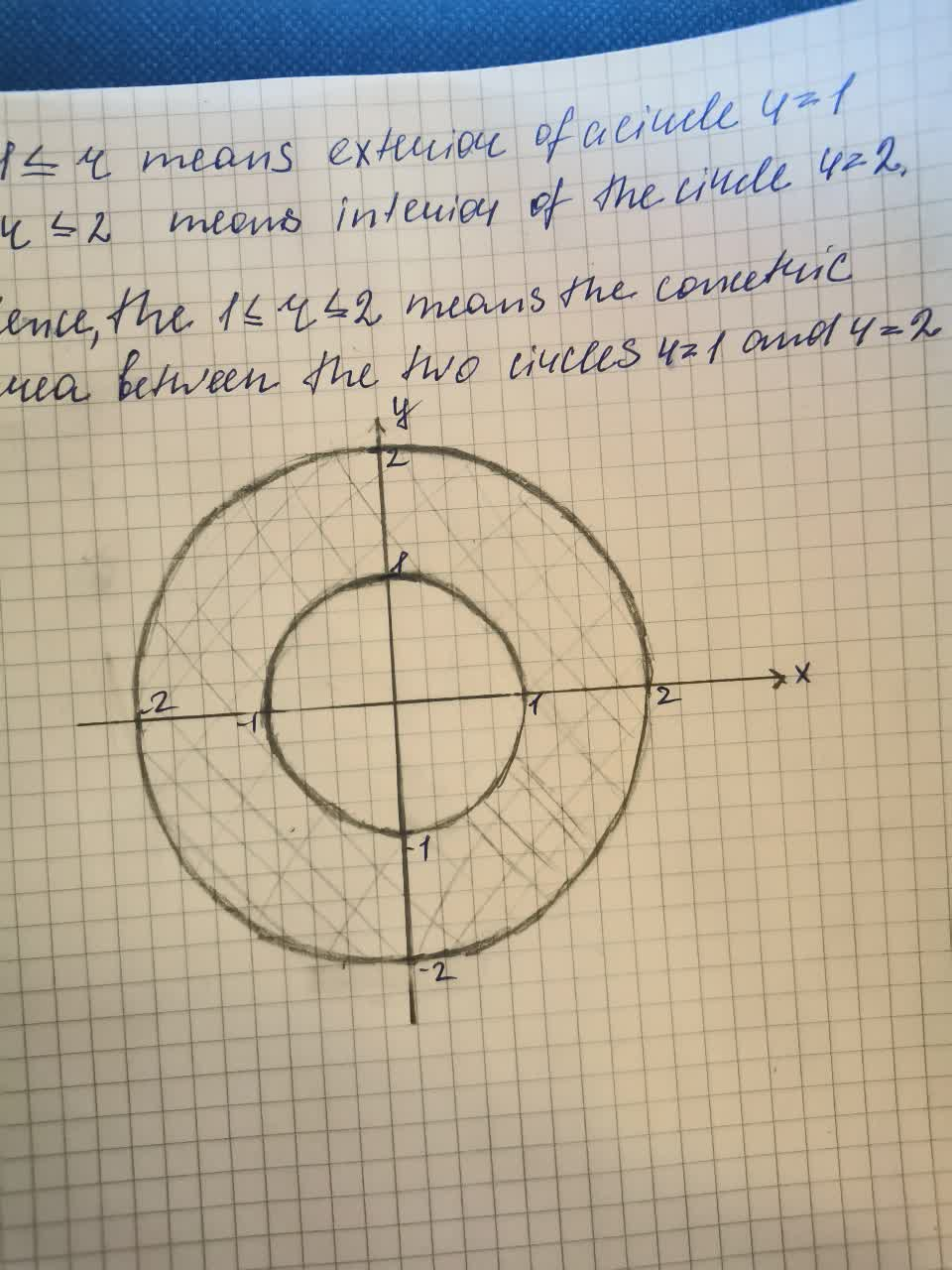# Graph the sets of points whose polar coordinates satisfy the equations and inequalitiesReggie 2021-08-21 Answered
Graph the sets of points whose polar coordinates satisfy the equations and inequalities $1\le r\le 2$
You can still ask an expert for help

• Questions are typically answered in as fast as 30 minutes

Solve your problem for the price of one coffee

• Math expert for every subject
• Pay only if we can solve ithosentak

Now we have